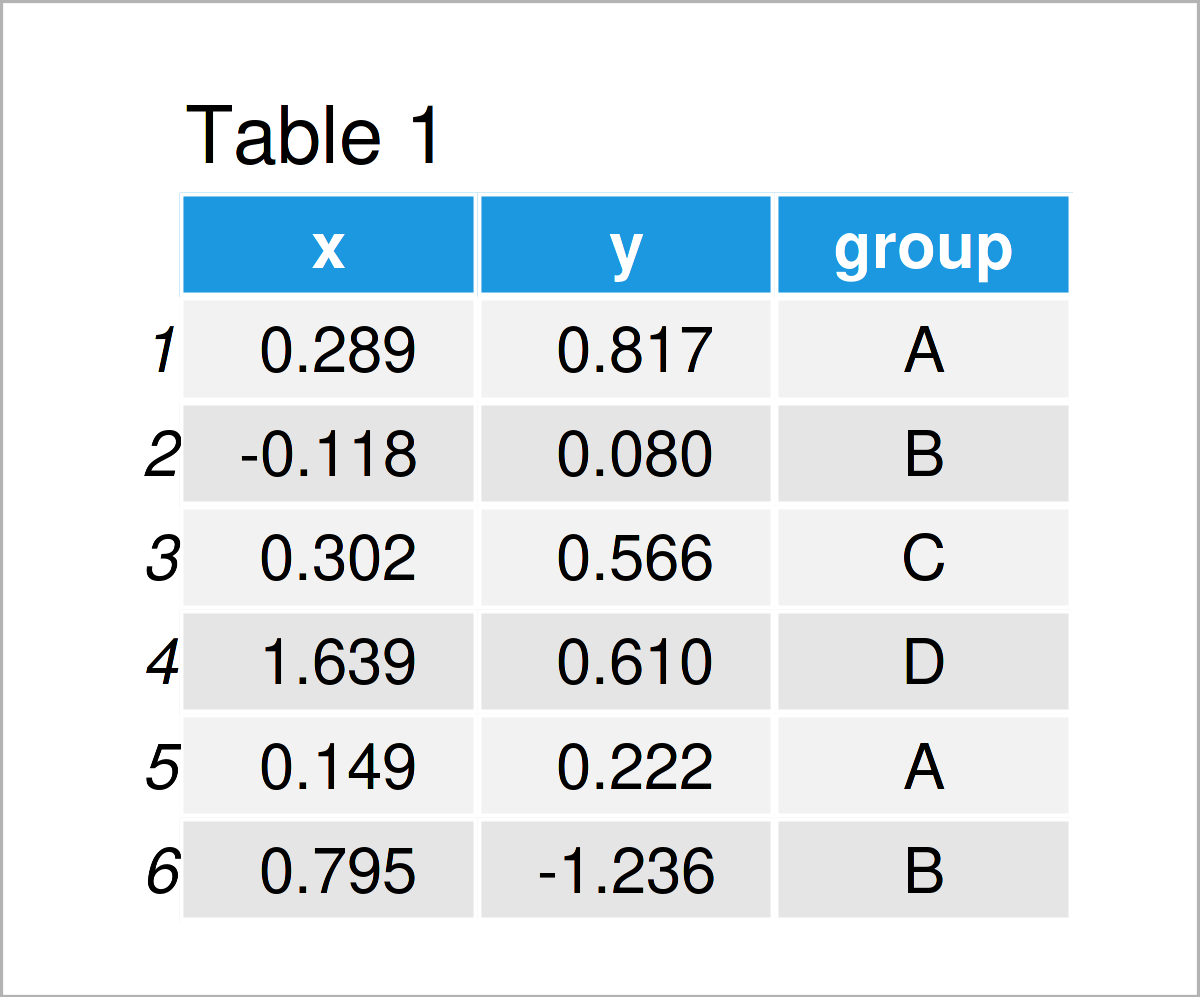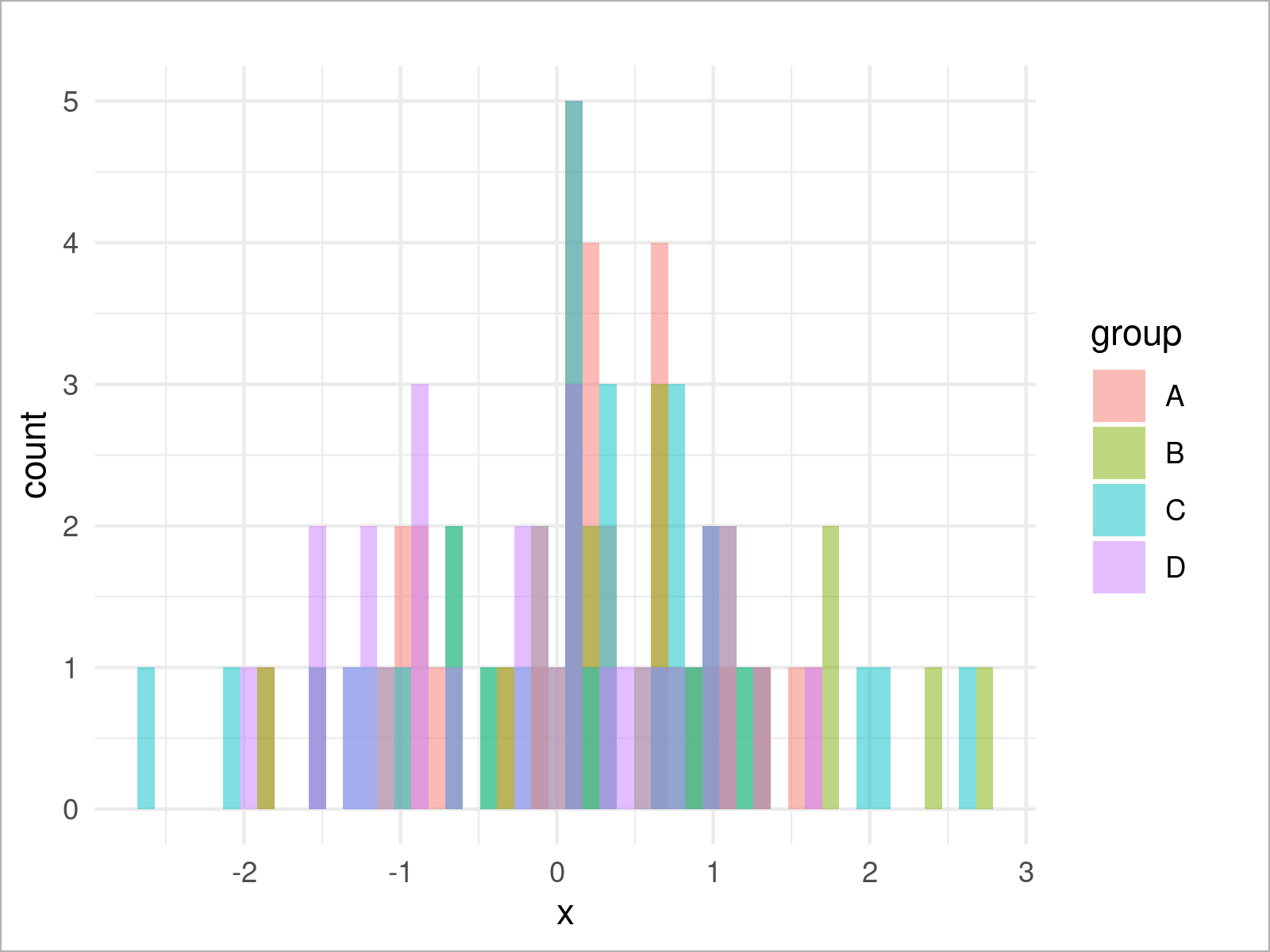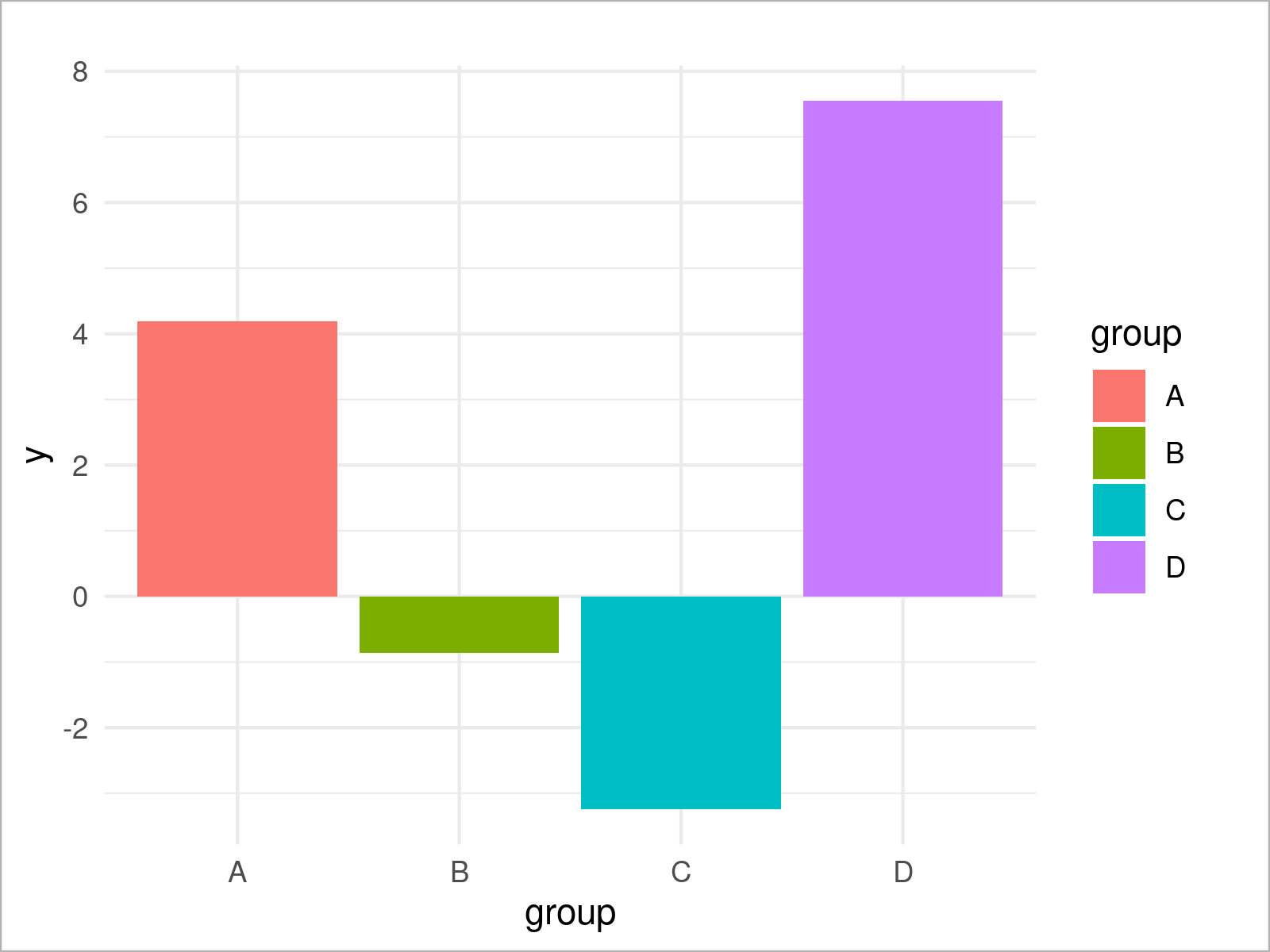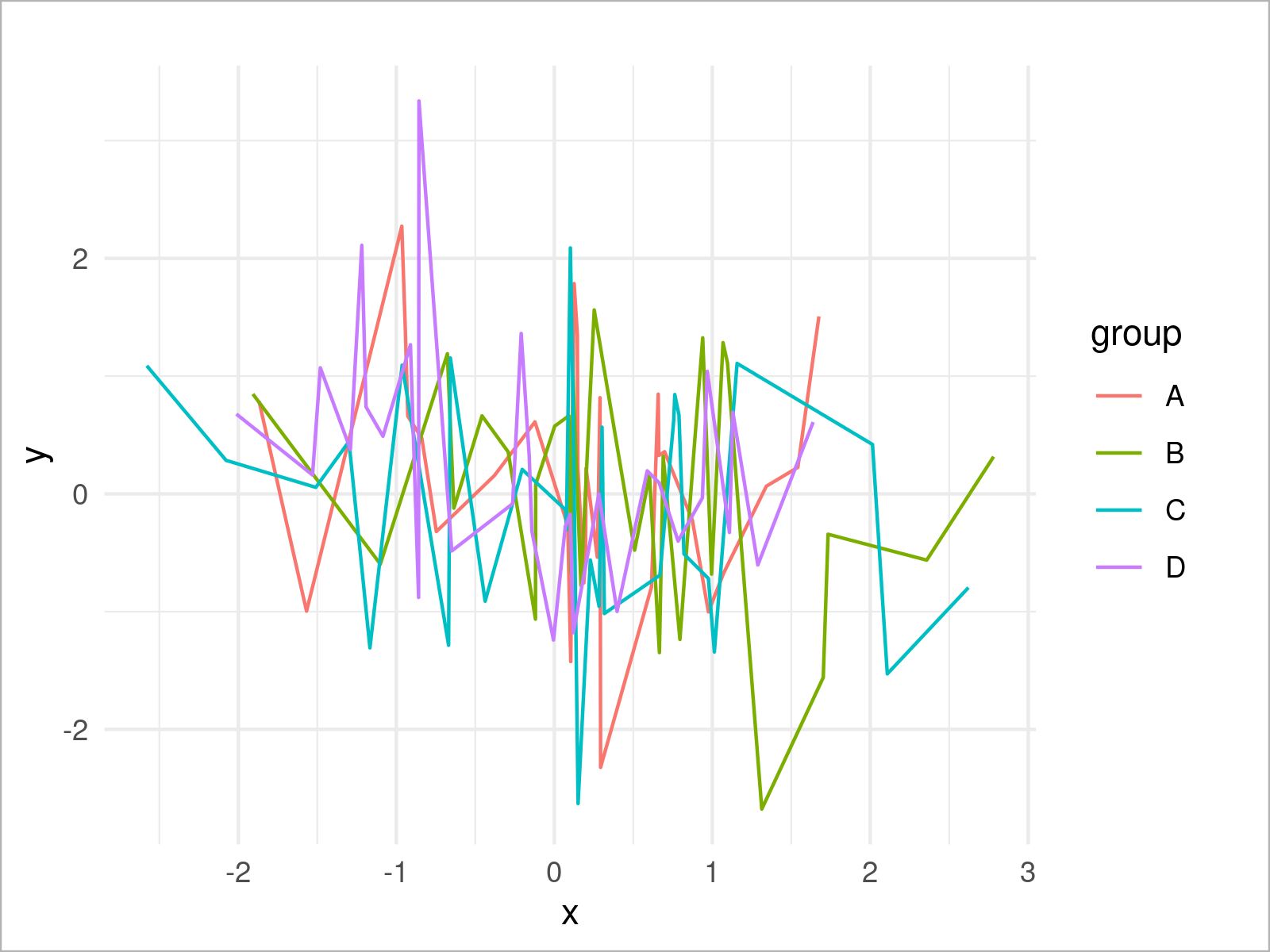# theme_minimal ggplot2 Theme in R (6 Examples)

In this post you’ll learn how to switch the ggplot2 theme to the theme_minimal in R programming.

So now the part you have been waiting for – the exemplifying R syntax.

## Example Data & Packages

I use the following data as basement for this R tutorial:

```set.seed(1171665)                          # Set random seed
data <- data.frame(x = rnorm(120),         # Create example data frame
y = rnorm(120),
group = LETTERS[1:4])Table 1 shows the first six rows of the exemplifying data – it is also visualized that our data contains three columns.

In order to use the commands and functions of the ggplot2 package, we also have to install and load ggplot2:

```install.packages("ggplot2")                # Install & load ggplot2 package
library("ggplot2")```

Let’s draw our data using a minimalistic style!

## Example 1: Draw ggplot2 Scatterplot Using theme_minimal()

```ggplot(data,                               # theme_minimal scatterplot
aes(x = x,
y = y,
col = group)) +
geom_point() +
theme_minimal()```## Example 2: Draw ggplot2 Density Plot Using theme_minimal()

```ggplot(data,                               # theme_minimal density plot
aes(x = x,
fill = group)) +
geom_density(alpha = 0.5) +
theme_minimal()```## Example 3: Draw ggplot2 Histogram Using theme_minimal()

```ggplot(data,                               # theme_minimal histogram
aes(x = x,
fill = group)) +
geom_histogram(alpha = 0.5,
position = "identity",
bins = 50) +
theme_minimal()```## Example 4: Draw ggplot2 Barchart Using theme_minimal()

```ggplot(aggregate(y ~ group, data, sum),    # theme_minimal barplot
aes(x = group,
y = y,
fill = group)) +
geom_bar(stat = "identity") +
theme_minimal()```## Example 5: Draw ggplot2 Boxplot Using theme_minimal()

```ggplot(data,                               # theme_minimal boxplot
aes(x = x,
fill = group)) +
geom_boxplot() +
theme_minimal()```## Example 6: Draw ggplot2 Line Plot Using theme_minimal()

```ggplot(data,                               # theme_minimal line plot
aes(x = x,
y = y,
col = group)) +
geom_line() +
theme_minimal()```## Video, Further Resources & Summary

If you are interested in data visualization in R and the functions of the ggplot2 package, you have to watch the following video, where I explain the ggplot2 package in much more detail (beginners & advanced users).

On Statistics Globe, you can also find tutorials on how to create other types of graphics such as scatterplots, density plots, barcharts, boxplots, histograms, and line plots using different ggplot2 themes:

Also, you may have a look at the introductions to other add-on packages that provide extra themes for ggplot2 plots:

Furthermore, you may have a look at the related R posts on this homepage. A selection of interesting articles is shown below:

This tutorial has demonstrated how to apply the theme_minimal function to modify the design and layout of a ggplot2 plot in the R programming language. If you have additional questions or comments, tell me about it in the comments.

Subscribe to the Statistics Globe Newsletter